# Javascript Tutorial: Higher-Order Function Examples

by Ridwan Fadilah, updated on May 21, 2020#### Another Javascript examples of Higher-Order Function that often to use such as filter, map, and reduce

Filter, map, and reduce is three of Higher-Order Function that often uses. The three functions of these is a prototype of the array data type. Although, these functions could contain miscellaneous such as an array of integer, string, or may array of objects.

## Filter

By using a filter() method, the method will return a new array with elements that pass the filtering rule (provide as a function).

For example, I have an array that contains some random numbers. I want to find the numbers that greater or equal to 3 by using the higher-order function filter().

To do that, we can immediately call the array that will be filtered.

Example:

``````� � <!DOCTYPE html>
� � <html lang="en">

� � � � <title>Higher Order Function</title>

� � <body>
� � � � <!-- Filter -->
� � � � <script>
� � � � � � const numbers = [-4, 9, 4, -1, -5, 8, 2, 3, -9];

� � � � � � const newNumbers = numbers.filter(function (n) {
� � � � � � � � return n >= 3;
� � � � � � });

� � � � � � console.log(newNumbers);
� � � � </script>
� � </body>

� � </html>``````

result: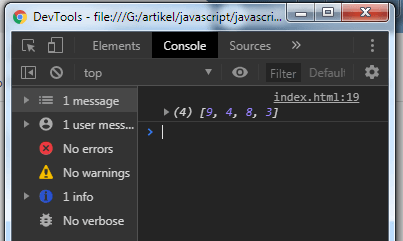We can also use an arrow function for the example above to make it simply:

``````� � const newNumbers = numbers.filter(n => n >= 3);
� � console.log(newNumbers);``````

That will return the same result.

## Map

We will map all the elements within an array by using a new function. For example, I want to have a new array whose contents are the result of every number multiplied by two.

Example:

``````� � <!DOCTYPE html>
� � <html lang="en">

� � � � <title>Higher Order Function</title>

� � <body>
� � � � <!-- Map -->
� � � � <script>
� � � � � � const numbers = [-4, 9, 4, -1, -5, 8, 2, 3, -9];

� � � � � � const newNumbers = numbers.map(n => n * 2);

� � � � � � console.log(newNumbers);
� � � � </script>
� � </body>

� � </html>``````

result: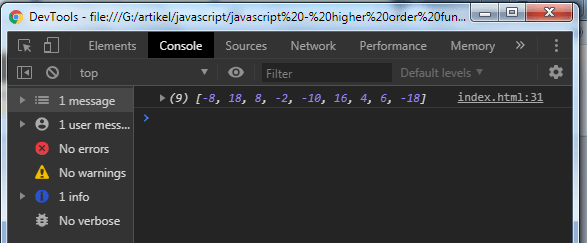This method will not change the elements of the numbers array:

``````� � <!DOCTYPE html>
� � <html lang="en">

� � � � <title>Higher Order Function</title>

� � <body>
� � � � <!-- Map -->
� � � � <script>
� � � � � � const numbers = [-4, 9, 4, -1, -5, 8, 2, 3, -9];

� � � � � � const newNumbers = numbers.map(n => n * 2);

� � � � � � console.log(numbers);
� � � � � � console.log(newNumbers);
� � � � </script>
� � </body>

� � </html>``````

result: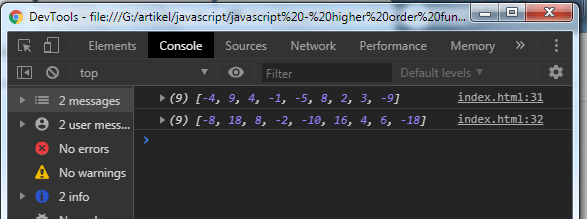## Reduce

The last of the higher-order function example is the reduce() function. That used to do something to the elements contained in the array.

In this case, I want to sum the elements contained in the numbers array.

Reduce are different from the others, they should have two parameters.

Example:

``````� � <!DOCTYPE html>
� � <html lang="en">

� � � � <title>Higher Order Function</title>

� � <body>
� � � � <!-- Reduce -->
� � � � <script>
� � � � � � const numbers = [-4, 9, 4, -1, -5, 8, 2, 3, -9];

� � � � � � const newNumber = numbers.reduce((accumulator, currentValue) => accumulator + currentValue);

� � � � � � console.log(newNumber);
� � � � </script>
� � </body>

� � </html>``````

result: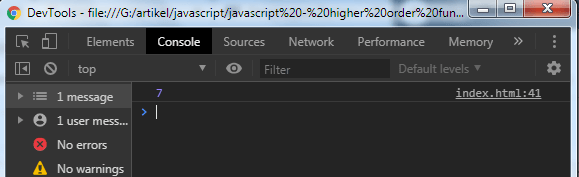By default, this function has the first value. The value is 0 (zero).
Here's the illustration of this function:

``� � 0 + -4 + 9 + 4 + -1 + -5 + 8 + 2 + 3 + -9``

You can set the first value of this function by using the following syntax:

``� � const newNumber = numbers.reduce((accumulator, currentValue) => accumulator + currentValue, 6);``

The illustration will be like this:

``� � 6 + -4 + 9 + 4 + -1 + -5 + 8 + 2 + 3 + -9``

The accumulator is the result of the process.
The currentValue is the element of the array that being looped.
However, you can set the name of the parameters as do you want, that's just an example.

## Chaining Method

With chaining, we can combine all of the Higher-Order Functions in one execution.

Example:

``````� � <!DOCTYPE html>
� � <html lang="en">

� � � � <title>Higher Order Function</title>

� � <body>
� � � � <!-- Chaining -->
� � � � <script>
� � � � � � const numbers = [-4, 9, 4, -1, -5, 8, 2, 3, -9];

� � � � � � const total = numbers.filter(n => n > 3) // will return 9, 4, 8
� � � � � � � � .map(n => n * 2) // will return 18, 8, 16
� � � � � � � � .reduce((accum, current) => accum + current); // will return 42

� � � � � � console.log(total);
� � � � </script>
� � </body>

� � </html>``````

result: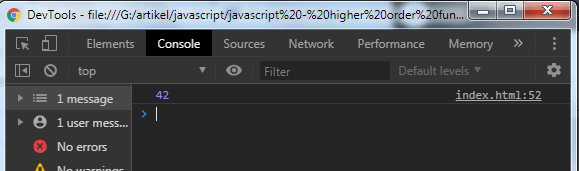All done. That's the little examples of the Higher-Order Functions. Everything you see in this tutorial is just a basic. You can find the full source code of these examples on our GitHub.